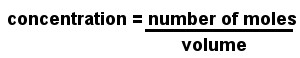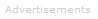# Definition of Molarity

What is Molarity?

Molarity is used to express the concentration of a solution. Also known as molar concentration, molarity is the number of moles of solute (the material dissolved) per liter of solution.The units of molarity are moles per cubic decimeter, written mol dm-3 or simply M. The cubic decimeter is identical to the liter; in many older textbooks you will find concentrations are written in moles per liter, written mol l-1.

2.0 mol dm-3 or 2.0 mol l-1 or 2.0 M all represent the same concentration.

In the Past

Once it was normal for chemists to quote concentrations as (weight of solute)/(volume). However, the mole is now the most common way in chemistry of expressing the quantity of a substance, therefore molar concentrations are nearly always used.

Note:

Be careful not to confuse molality and molarity. Molality's units are often represented by a lower case "m" whereas molarity's are often represented by an upper case "M".Search the Dictionary for More Terms